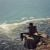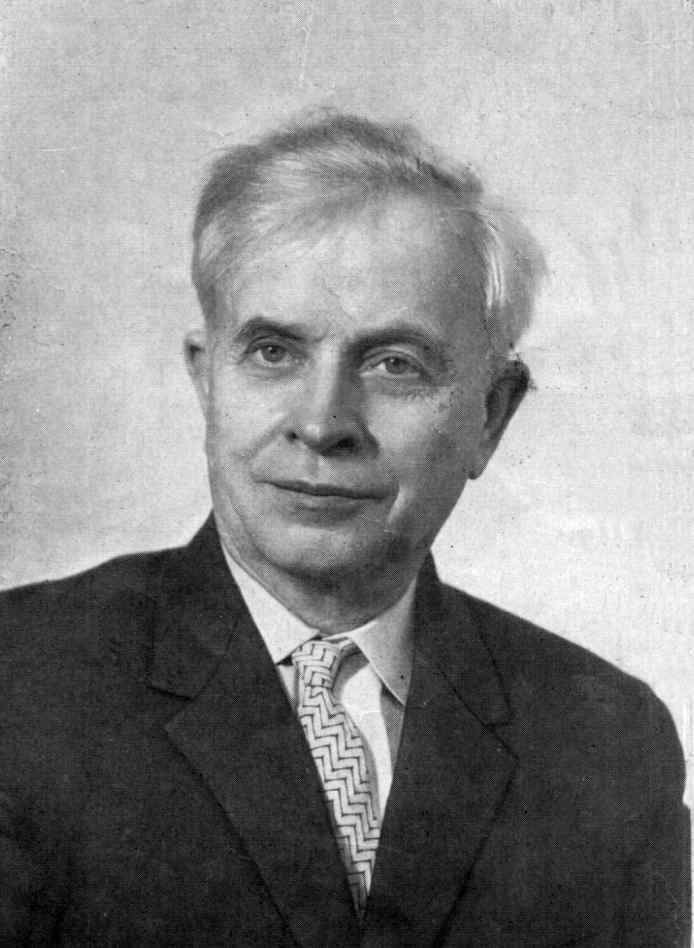# Andrey Markov, Jr

Updated on
 Name  Andrey Jr.Role  Mathematician Parents  Andrey MarkovBooks  Theory of AlgorithmsDied  October 11, 1979, Moscow, RussiaUncles  Vladimir Andreevich Markov

Andrey Andreyevich Markov Jr. (Russian: Андре́й Андре́евич Ма́рков; St. Petersburg, September 22, 1903 – Moscow, October 11, 1979) was a Soviet mathematician, the son of the Russian mathematician Andrey Andreyevich Markov Sr, and one of the key founders of the Russian school of constructive mathematics and logic. He made outstanding contributions to various areas of mathematics, including differential equations, topology, mathematical logic and the foundations of mathematics.

His name is in particular associated with Markov's principle, Markov's rule, and Markov algorithm. An important result which he proved in 1947 was that the word problem for semigroups was unsolvable; Emil Post obtained the same result independently at about the same time. In 1953 he became a member of the Communist Party.

In 1960, Markov obtained fundamental results showing that the classification of four-dimensional manifolds is undecidable (no general algorithm exists for distinguishing two arbitrary manifolds with four or more dimensions). This is because four dimensional manifolds have sufficient flexibility to allow us to embed any algorithm within their structure, so that classification of all four-manifolds would imply a solution to Turing's halting problem. This result has profound implications for the limitations of mathematical analysis.

Similar Topics
Andrey Markov
Rod Canion
Ron Perkins
Topics